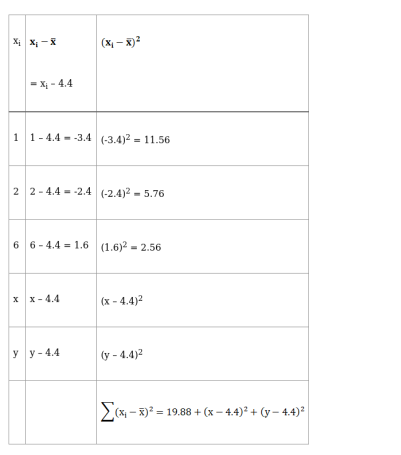# The mean and variance of five observations are 4.4 and 8.24 respectively.

Question:

The mean and variance of five observations are 4.4 and 8.24 respectively. If three of these are 1, 2 and 6, find the other two observations.

Solution:

Given: Mean of 5 observations = 4.4

and Variance of 5 observations $=8.24$

Let the other two observations be $x$ and $y$

$\therefore$, our observations are $1,2,6, \mathrm{x}$ and $\mathrm{y}$

Now, we know that,

Mean $(\overline{\mathrm{x}})=\frac{\text { Sum of observations }}{\text { Total number of observations }}$

$4.4=\frac{1+2+6+x+y}{5}$

$\Rightarrow 5 \times 4.4=9+x+y$

$\Rightarrow 22-9=x+y$

$\Rightarrow 13=x+y$

or $x+y=13 \ldots$ (i)

Also,

Variance = 8.24

Variance, $\sigma^{2}=\frac{\sum\left(\mathrm{x}_{\mathrm{i}}-\overline{\mathrm{x}}\right)^{2}}{\mathrm{n}}$So,

Variance, $\sigma^{2}=\frac{\sum\left(\mathrm{x}_{\mathrm{i}}-\overline{\mathrm{x}}\right)^{2}}{\mathrm{n}}$

$8.24=\frac{19.88+(x-4.4)^{2}+(y-4.4)^{2}}{5}$

$\Rightarrow 41.2=19.88+\left(x^{2}+19.36-8.8 x\right)+\left(y^{2}+19.36-8.8 y\right)$

$\Rightarrow 41.2-19.88=x^{2}+19.36-8.8 x+y^{2}+19.36-8.8 y$

$\Rightarrow 21.32=x^{2}+y^{2}+38.72-8.8(x+y)$

$\Rightarrow x^{2}+y^{2}+38.72-8.8(13)-21.32=0[$ from (i) $]$

$\Rightarrow x^{2}+y^{2}+17.4-114.4=0$

$\Rightarrow x^{2}+y^{2}-97=0$

$\Rightarrow x^{2}+y^{2}=97 \ldots$ (ii)

From eq. (i)

x + y = 17.4

Squaring both the sides, we get

$(x+y)^{2}=(13)^{2}$

$\Rightarrow x^{2}+y^{2}+2 x y=169$

$\Rightarrow 97+2 x y=169[$ from (ii)]

$\Rightarrow 2 x y=169-97$

$\Rightarrow 2 x y=72$

$\Rightarrow x y=36$

$\Rightarrow \mathrm{x}=\frac{36}{\mathrm{y}}$ …(iii)

Putting the value of x in eq. (i), we get

$x+y=13$

$\Rightarrow \frac{36}{y}+y=13$

$\Rightarrow \frac{36+y^{2}}{y}=13$

$\Rightarrow y^{2}+36=13 y$

$\Rightarrow y^{2}-13 y+36=0$

$\Rightarrow y^{2}-4 y-9 y+36=0$

$\Rightarrow y(y-4)-9(y-4)=0$

$\Rightarrow(y-4)(y-9)=0$

$\Rightarrow y-4=0$ and $y-9=0$

$\Rightarrow y=4$ and $y=9$

For y = 4

$x=\frac{36}{y}=\frac{36}{4}=9$

Hence, x = 9, y = 4 are the remaining two observations

For y = 9

$x=\frac{36}{y}=\frac{36}{9}=4$

Hence, x = 4, y = 9 are the remaining two observations

Thus, remaining two observations are 4 and 9.